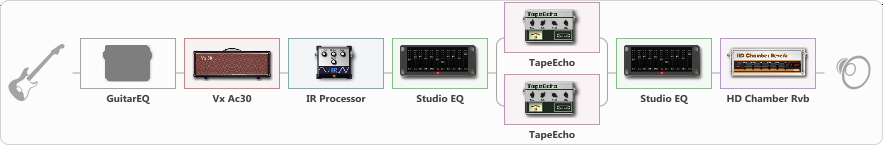# Last Date - The Spotnicks (Bo Winberg)

Discussion in 'ToneLib-GFX presets' started by eddy.grand, Jul 10, 2020.

1. Last Date - The Spotnicks (Bo Winberg)
Preset name: Last Date - The Spotnicks

Stratocaster, Middle-PU.
Hope, You like it.

Effects chain:Effect: "GuitarEQ" (Dynamics / Filter), active - "no"
{

"160 Hz" = 0
"400 Hz" = 0
"800 Hz" = 0
"1.6 kHz" = 0
"3.2 kHz" = 0
"6.4 kHz" = 0
"12 kHz" = 0
"Level (dB)" = 0
}

Effect: "Vx Ac30" (Amp simulators), active - "yes"
{

"Gain" = 20
"Bass" = 2
"Middle" = 90
"Treble" = 39
"Presence" = 50
"Master" = 80
"Level (dB)" = 10
}

Effect: "IR Processor" (Cabinets), active - "yes"
{

"IR" = Vox AC30
"Low Cut (Hz)" = 57
"Hi Cut (kHz)" = 7.5
"Mix" = 100
"Level (dB)" = 0
}

Effect: "Studio EQ" (Dynamics / Filter), active - "yes"
{

"31 Hz" = -15
"62 Hz" = -15
"125 Hz" = -7
"250 Hz" = 2
"500 Hz" = 3
"1 kHz" = -3
"2 kHz" = 5
"4 kHz" = 6
"8 kHz" = 6
"16 kHz" = -4
"Level (dB)" = 0
}

Effect: "Splitter" (Dynamics / Filter), active - "yes"
{

"A-Bypass" = Off
"A-Pan" = 0
"A-Level" = 55
"B-Bypass" = Off
"B-Pan" = 0
"B-Level" = 55
'A' branch:
{

Effect: "TapeEcho" (Delay), active - "yes"
{

"Time" = 100
"Feedback" = 0
"Tone" = 47
"LoDamp" = 35
"Mix" = 57
}
}
'B' branch:
{

Effect: "TapeEcho" (Delay), active - "yes"
{

"Time" = 200
"Feedback" = 58
"Tone" = 65
"LoDamp" = 19
"Mix" = 72
}
}
}

Effect: "Studio EQ" (Dynamics / Filter), active - "yes"
{

"31 Hz" = -15
"62 Hz" = -13
"125 Hz" = 4
"250 Hz" = 2
"500 Hz" = -6
"1 kHz" = -1
"2 kHz" = 8
"4 kHz" = 6
"8 kHz" = -12
"16 kHz" = -12
"Level (dB)" = 0
}

Effect: "HD Chamber Rvb" (Reverberation), active - "yes"
{

"Time" = 5.1
"PreLPF" = 100
"PreDelay" = 202
"HiDamp" = 0
"LoGain" = 12.0
"Mix" = 35
}

Note: You will need to download and install the ToneLib-GFX software to use the preset.

#### Attached Files:

• ###### Last_Date_-_The_Spotnicks_Bo_Winberg.tlgfx
File size:
1.4 KB
Views:
9,497
Last edited: Jul 10, 2020
2. Thanks IMKNSHI!

3. Thank you, rrv!

4. Many thanks Mic Vanessche!

5. Thanks Pavel Saidl.

6. Many Thanks, magichornbeam.

Last edited: Mar 29, 2021
7. Thank you Farkhad Tulegen.

8. Thank you, oblnsky!

9. Thanks Dmitry Borisov!

10. Thank you SEMENOV!

11. Thanks Vladimir L.!

12. Thank you athra maroo!

13. Thanks propalnenadolgo!